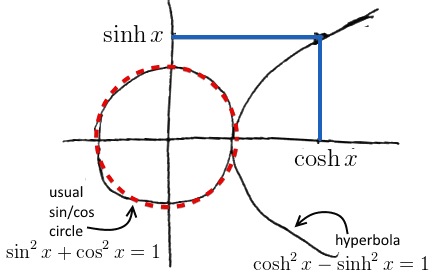# ML Wiki

## Hyperbolic Trigonometric Functions

Hyperbolic trigs are analogs of usual Trigonometric Functions

• $\cosh x = \cfrac{e^x + e^{-x}}{2}$
• $\sinh x = \cfrac{e^x - e^{-x}}{2}$
• $\tanh x = \cfrac{\sinh x}{\cosh x} = \cfrac{e^x - e^{-x}}{e^x + e^{-x}}$(source: )

• $\cosh^2 x - \sinh^2 x = 1$
•Taylor Expansion:

• $\cosh x = \cfrac{e^x + e^{-x}}{2} = \cfrac{1}{2}\, e^x + \cfrac{1}{2}\, e^{-x} = \ ...$
• $... \ = \cfrac{1}{2}\, \left(1 + x + \cfrac{1}{2!}\, x^2 + \cfrac{1}{3!}\, x^3 + \ ... \ \right) + \cfrac{1}{2}\, \left(1 - x + \cfrac{1}{2!}\, x^2 - \cfrac{1}{3!}\, x^3 + \ ... \ \right) = \ ...$
• odd degree terms cancel out
• $... \ = 1 + \cfrac{1}{2!}\, x^2 + + \cfrac{1}{4!}\, x^4 + \ ... \ = \sum\limits_{k=0}^\infty \cfrac{x^2}{(2k)!}$
• co we have even powers, like for $\cos x$, just without $(-1)^k$ term
• same for $\sinh x = \cfrac{1}{2}\, e^x - \cfrac{1}{2}\, e^{-x} = \sum\limits_{k=0}^\infty \cfrac{x^{2k + 1}}{(2k+1)!}$
• if we Taylor expand $e^x$, even powers cancel out, and we're left only with odd powers
• just like usual $\sin x$, but without alternating sing

## Derivatives

• $\cfrac{d}{dx}\, \sinh x = \cosh x$
• $\cfrac{d}{dx}\, \cosh x = \sinh x$

Can show that with Taylor expansions:

• $\cfrac{d}{dx}\, \sinh x = \cfrac{d}{dx} \sum\limits_{k=0}^\infty \cfrac{x^{2k + 1}}{(2k+1)!} = \sum\limits_{k=0}^\infty (2k+1)\, \cfrac{x^{2k}}{(2k+1)!} = \sum\limits_{k=0}^\infty \cfrac{x^{2k}}{(2k)!} = \cosh x$
• same with $\cosh x$